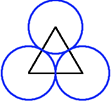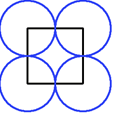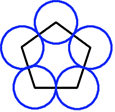#### You may also like### Overturning Fracsum

Solve the system of equations to find the values of x, y and z: xy/(x+y)=1/2, yz/(y+z)=1/3, zx/(z+x)=1/7### Building Tetrahedra

Can you make a tetrahedron whose faces all have the same perimeter?### Rudolff's Problem

A group of 20 people pay a total of £20 to see an exhibition. The admission price is £3 for men, £2 for women and 50p for children. How many men, women and children are there in the group?

# Polycircles

##### Age 14 to 16 Challenge Level:For any regular polygon it is always possible to draw circles with centres at the vertices of the polygon, and radii equal to half the length of the edges, to form a "polycircle" in which each circle just touches its neighbours.

Investigate and explain what happens in the case of non-regular polygons. Is it always possible to construct three circles with centres at the vertices of the triangle so that the circles just touch?

Try a numerical example, say the 3 points give a triangle of sides 12, 15 and 13. Find the radii of the circles.

Now use the same method when the sides of the triangle are $a$, $b$ and $c$.

Generalise still more! What about 4 circles around a quadrilateral? 5 circles around a pentagon? $n$ circles around an n-gon?

You may wish to use GeoGebra to explore.